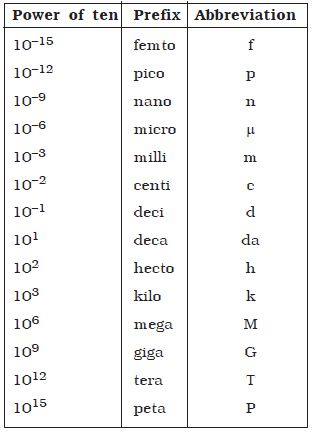Physics

# Expressing Larger and Smaller Physical Quantities

It is easier to express larger and smaller units of the same physical quantity. In the metric (SI) system these are related to the fundamental unit in multiples of 10 or 1/10. Thus 1 km is 1000 m and 1 mm is 1/1000 metre. Table lists the standard SI prefixes, their meanings and abbreviations. In order to measure very large distances, the following units are used.(i) Light year Light year is the distance travelled by light in one year in vacuum.

Distance travelled = velocity of light x 1 year

So, 1 light year = 3 x 108 m s-1 x 1 year tin seconds)

= 3 x 108 x 365.25 x 24 x 60 x 60

= 9.467 x 1015 m

So, 1 light year = 9.467 x 1015 m

(ii) Astronomical unit Astronomical unit is the mean distance of the centre of the Sun from the centre of the Earth.

1 Astronomical unit (A11) = 1.496 x 1011 m.